Question

According to the reaction, below, how many grams of aluminum are needed to react fully with 100 grams of sulfur? 2AL + 3s – Al2S3

1.Answer: 56.2 g of Aluminium is needed to react fully with 100 grams of sulphur.

Explanation:

To calculate the moles :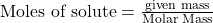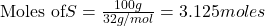The balanced chemical equation is: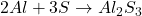According to stoichiometry :

3 moles of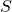require = 2 moles of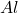Thus 3.125 moles ofwill require=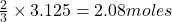ofMass of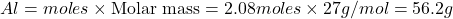Thus 56.2 g of Aluminium is needed to react fully with 100 grams of sulphur.